Next: About this document ... Up: lab_template Previous: lab_template

Subsections

# MA 1024 lab 1 - Parametric Curves and Vector-valued Functions in the Plane

## Purpose

The purpose of this lab is to introduce you to curve computations using Maple for parametric curves and vector-valued functions in the plane.

## Getting Started

To assist you, there is a worksheet associated with this lab that contains examples and even solutions to some of the exercises. You can copy that worksheet to your home directory with the following command, which must be run in a terminal window, not in Maple.

cp ~bfarr/Curves2D_start.mws ~


You can copy the worksheet now, but you should read through the lab before you load it into Maple. Once you have read to the exercises, start up Maple, load the worksheet Curves2D_start.mws, and go through it carefully. Then you can start working on the exercises.

## Background

By parametric curve in the plane, we mean a pair of equationsandfor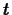in some interval. A vector-valued function in the plane is a function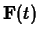that associates a vector in the plane with each value ofin its domain. Such a vector valued function can always be written in component form as follows,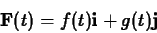where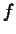and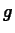are functions defined on some interval. From our definition of a parametric curve, it should be clear that you can always associate a parametric curve with a vector-valued function by just considering the curve traced out by the head of the vector asvaries over the interval. However, there are lots of situations where a vector-valued function is more appropriate. This happens most often when the quantity described by the function is a vector, for example, a force or a displacement. However, there can be situations when the quantity is not a vector, but it is convenient to think of it as a vector to simplify the notation or the calculations. The idea to keep in your mind is that one of the motivations of vector notation is to simplify and clarify calculations or other manipulations.

Many applications of calculus to engineering or science involve parametric curves or vector valued functions, including the examples listed below.

• The kinematics of mechanisms, like internal combustion engines.
• Population models involving more than one species.
• Chemical kinetics in systems with more than one chemical species.
• Forced or transient responses of electrical circuits.

For the applications we will deal with, we only need to consider curvilinear motion of a point in the plane, That is, we have a vector-valued functionthat gives the position at timeof a moving point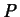in the plane. The velocity of this point is given by the derivative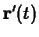and the acceleration is given by the second derivative,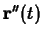. If the velocity,, is not the zero vector, then it is clear from the way it is defined thatis a vector that is tangent to the curve at the point. A simple example of curvilinear motion is when the velocity is constant. That is, there is a fixed vector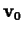such that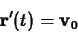In this case, the motion is along a straight line, withgiven by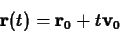whereis the position at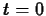.

In many applications of curvilinear motion, we need to know the magnitude of the velocity, or the speed. This is easy to compute - just take the magnitude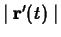. Ifandare the component functions of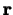, then the speed is given by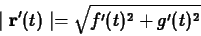Using our idea of the parametric curve associated withand recalling the definition of arc length, we arrive at a different interpretation of speed as the rate of change of arc length, or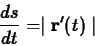where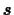is arc length. This should make sense, if you recall that speed is the rate of change of distance with time and arc length is distance measured along a curve.

If the speed is not zero for any value ofin the interval, then it is possible to define a unit vector,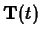that is tangent to the curve as follows.Using this definition, you can write the velocity in the following form.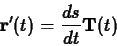Now, it is natural for the student to ask why one would want to do this. Computing the velocity using the right hand side of the equation above is certainly more difficult than taking the derivative of, so this equation is not really useful from a computational viewpoint. The answer to this is that the unit tangent vector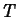at a particular point on the curve is an intrinsic property of the curve, at least up to a sign change. To use an analogy, think of the curve as being a one-way road. Different drivers can drive at different speeds, but at a particular point on the road, each car is pointed in the same direction, i.e. the direction of the unit tangent vector at that point. Now suppose that the road allows traffic in both directions. If you think about it a little, it should be clear that if the tangent vector at a particular point on the road for traffic going one direction is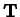, then the tangent vector at the same point for traffic going the opposite direction is.

Now suppose, to carry our analogy a little further, that you wanted to analyze the acceleration of a car as it traversed the road. On sections where the road is straight, acceleration depends only on whether the car is speeding up or slowing down. In a curve, however, there is an additional acceleration, called the centripetal acceleration, that is needed to keep the car on the road. The magnitude of this acceleration depends on the speed of the car and the sharpness of the curve. It turns out that you can quantify the sharpness of a curve with an intrinsic property of the curve called the curvature, usually denoted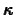, defined by the following equation.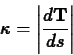That is, the curvature is the magnitude of the rate of change of the tangent vectorwith respect to arc length. For example, the curvature of a straight line is zero and it can be shown that the curvature of a circle of radiusis the same for every point on the circle and is given by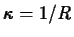.

If the curvature is never zero for a particular curve, then we can define another intrinsic property of curve, the unit normal vector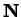by the following equation.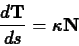It can be shown that at each point on the curve the vectordefined by this equation is a unit vector that is always perpendicular to the tangent vectorat that point. Furthermore, the unit normal vectoralways points in the direction of the centripetal acceleration required to keep a particle moving on the curve. One way to see this is to compute the acceleration by differentiating both sides of the equationfor the velocity and using the chain rule and the definition of the curvature and the normal vector to obtain the following important equation.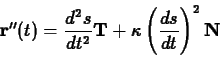To see why this equation is important, recall that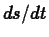is the speed, so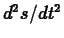is the rate of change of the speed. In our analogy of a car driving on a road, this term measures whether the car is speeding up or slowing down. Because this component of the acceleration acts in the direction of the tangent vector it is called the tangential acceleration, denoted with the symbol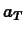. The component of the acceleration in the direction of the normal vector is called the normal acceleration, denoted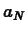.

Computing these intrinsic properties of a curve is generally not an easy task. The Getting started worksheet for this lab describes commands from the CalcP package that simplify these calculations and provides examples for you to work from.

## Exercises

1. Consider the simple function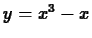for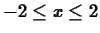.
1. Show that the graph of the parametric curve given by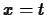,for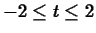is the function. Asincreases, is the motion on the graph from right to left or from left to right?
2. Show that the graph of the parametric curve given by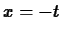,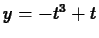foris also the functionbut that the direction of motion is opposite to the parametrization in the previous part of this exercise.

2. Consider the parametric curve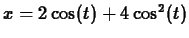,.
1. Plot the graph of this parametric curve for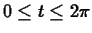. Identify the points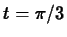andon your graph. This is probably best done by hand on the printed copy.
2. Find the maximum and minimum values of the speed.
3. Explain why the graph you plotted in the first part of this exercise is the cardiod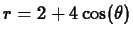. What does the parametercorrespond to?

3. Find a parametric description for the three-leafed rose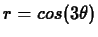. That is, find functions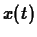and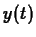such that the graph of the parametric curve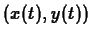is the three-leafed rose.

4. Consider the parametric curve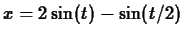,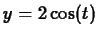for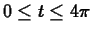.
1. For which value(s) ofis the speed a minimum?
2. For which value(s) ofis the curvature a maximum?
3. For which value(s) ofis the tangential acceleration a maximum?
4. For which value(s) ofis the normal acceleration a maximum?Next: About this document ... Up: lab_template Previous: lab_template
Dina Solitro
2001-03-20